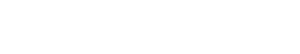# Lemmatization

A lemma (plural lemmas or lemmata) is the canonical form, dictionary form, or citation form of a set of words. A lemma is often used as the headword or main entry word in dictionaries. When a word is inflected, its lemma is the uninflected form of the word. For example, the lemma of “went” is “go”, and the lemma of “running” is “run”.

In the text analytics industry, lemmatization is the process of grouping together the different inflected forms of a word so that they can be analyzed as a single item. For example, the words “goes”, “going”, and “went” would all be grouped together under the lemma “go”.

Lemmatization is often used to improve the accuracy of text analytics algorithms by reducing the dimensionality of the data. It can also be used to simplify the storage and retrieval of data.

Lemmatization of adjectives is the process of grouping together the different inflected forms of an adjective so that they can be analyzed as a single item. For example, the words “red”, “redder”, and “reddest” would all be grouped together under the lemma “red”.

Lemmatization of adverbs is the process of grouping together the different inflected forms of an adverb so that they can be analyzed as a single item. For example, the words “quickly”, “more quickly”, and “most quickly” would all be grouped together under the lemma “quick”.

## Lemmatization for Verbs

Lemmatization of verbs is the process of grouping together the different inflected forms of a verb so that they can be analyzed as a single item. For example, the words “speak”, “speaks”, “speaking”, and “spoke” would all be grouped together under the lemma “speak”.

## Lemmatization for Noun Groups

Lemmatization of noun groups is the process of grouping together the different inflected forms of a group of related nouns so that they can be analyzed as a single item. For example, the words “dog”, “dogs”, and “dogma” would all be grouped together under the lemma “dog”.

## Lemmatization for Proper Nouns

Lemmatization of proper nouns is the process of grouping together the different inflected forms of a proper noun so that they can be analyzed as a single item. For example, the word “John” could be grouped together with the word “Johnson” under the lemma “John”.

## Lemmatization for Pronouns

Lemmatization of pronouns is the process of grouping together the different inflected forms of a pronoun so that they can be analyzed as a single item. For example, the words “I”, “me”, “we”, and “us” would all be grouped together under the lemma “I”.

## Lemmatization vs Stemming

Lemmatization and stemming are similar in that they both reduce the dimensionality of data. However, lemmatization does this by grouping together different inflected forms of a word, while stemming reduces a word to its root form.

For example, the word “running” could be reduced to its stem “run”. However, the word “runs” would also be reduced to the stem “run”, even though it has a different meaning. In contrast, lemmatization would group together the words “running”, “runs”, and “ran” under the lemma “run”, but it would not group together the word “run”.Unlock the power of actionable insights with AI-based natural language processing.